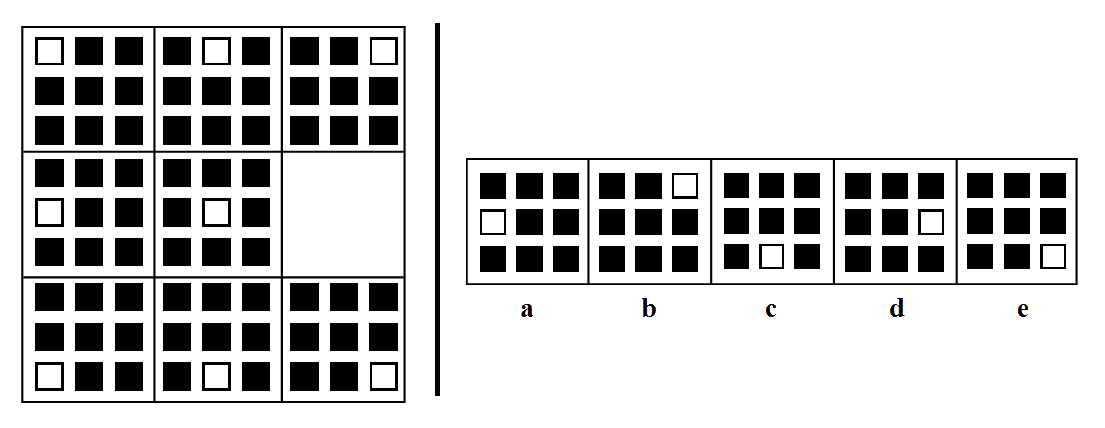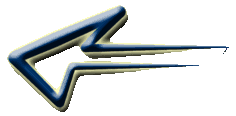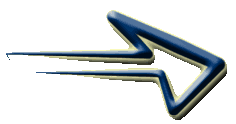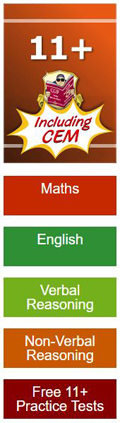# Type 6 - 'Missing Square'

With this type of question you are given a grid of either four or nine squares to the left of a vertical line. All but one of these squares contain symbols or shapes. To the right of the vertical line are five boxes labelled 'a' to 'e' containing symbols which are the possible answers.

You are required to determine which of the boxes to the right would best complete the grid of squares on the left.

Remember that the figures and diagrams consist of lines, shapes and shading/patterns. The shapes can shift in various ways. (rotate, reflect and transpose). The shapes can change in many ways. (increase/decrease, add and copy). They can consist of a movement, size or number sequence.Begin by looking at the symbols within the squares on the left and try to work out what the sequence is. In this case the symbols are a block of nine small squares. Within each block one square is white and the rest are black. The position of the white square forms a pattern which relates to the overall position in the grid.

Now you have discovered the pattern or sequence involved it is just a case of deciding what symbol from the selection of boxes on the right would complete the grid. In this case it would be 'd' because the white square is in the correct position to complete the pattern of the grid.

Practice working with Non Verbal Reasoning Type 6 questions with this
Non Verbal Reasoning Type 6 worksheet

##Non Verbal Type 6 worksheet